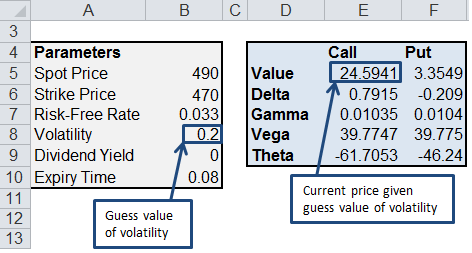# Implied Volatility

Implied volatility is the volatility that matches the current price of an option, and represents current and future perceptions of market risk. This is in contrast to the normal definition of volatility, which is backwards-facing and is calculated from historical data (i.e. standard deviation of historical returns).

If traders expect the price of a stock to vary a lot, then its implied volatility (and Call and Put options) will trend upwards.  Implied volatilities often exceed their historic counterparts prior to a major announcement (such as an earnings announcement or a merger), and tend to the mean afterwards. For example, if the market is enthusiastic about a specific stock (perhaps due to a great earnings report), then a Call option will be expensive.  Accordingly, a covered Call is a good strategy.

Vega is  rate of change in the value of an option given a 1% change in volatility.  Hence knowing Vega prior to major announcements  is essential in correctly pricing an option.  Unless the price of a stock changes to reflect lower implied volatility, then puts/calls are expected to decline after a major announcement.

Some financial analysts consider implied volatility to be a price or value (rather than a statistical measure), given that it is directly derived from the transaction between a buyer-seller pair.

## Calculate Implied Volatility with Excel

Excel’s Goal Seek can be used to backsolve for the volatility of a European Option (priced using Black-Scholes) given the spot price, strike price, risk-free rate and time to expiration.  An example is given in the spreadsheet below (scroll to the bottom for the download link), but let’s go through a worked example first.

Calculate the implied volatility of a European option with a

• Spot Price of 490,
• Strike Price of 470,
• Risk-Free Rate of 0.033,
• Expiry time of 0.08,
• Call price of 30.

Step 1. In the spreadsheet, enter the Spot price, Strike price, risk free rate and Expiry time. Also, enter an initial guess value for the volatility (this will give you an initial Call price that is refined in the next step)Step 2.  Go to Data>What If Analysis>Goal Seek.  Set the Call value to 30 (cell E5 in the spreadsheet) by changing the volatility (cell B8 in the spreadsheet)You should find that volatility has been updated to 0.32 to reflect the desired Call price of 30.### 6 thoughts on “Implied Volatility”

1. Very interesting

I modified it so that it can be used on the Black formula of a cap.

Thanks a lot

2. can this be used for American options?

3. Great job!

How can I automated goal seek for calculating implied volatility of a list of option?
Thank you

4. Hi, This implied volatility sheet is a great help.
But, my problem is I want to back solve, I want the corresponding Underlying Rate when the Option price is “X” and the IV is known to be “Y”, the option type is European Put Option…
I am looking for Implied Stock Price.
Thanks & Regards,
Tejas